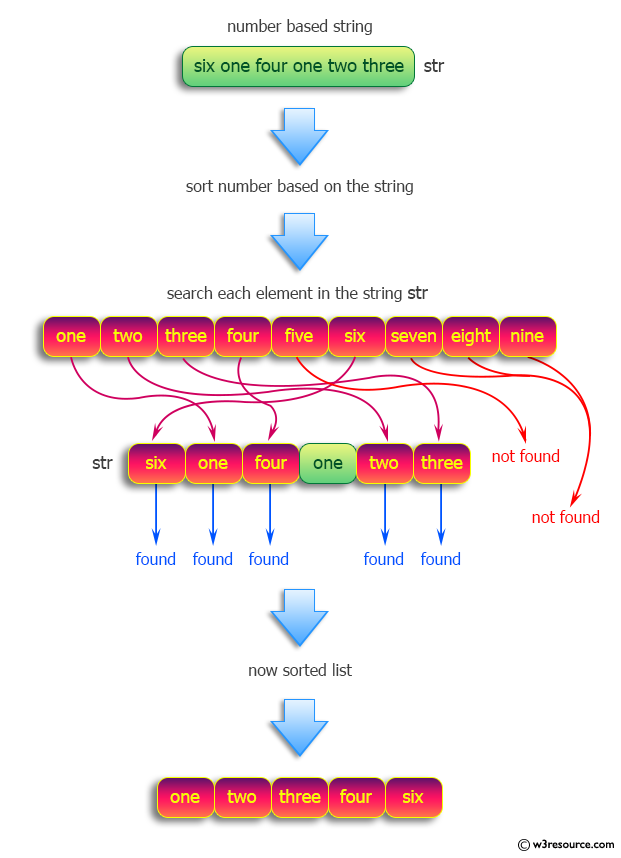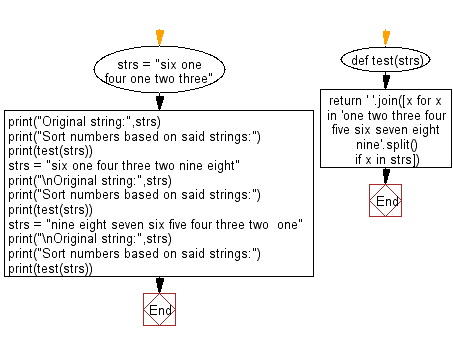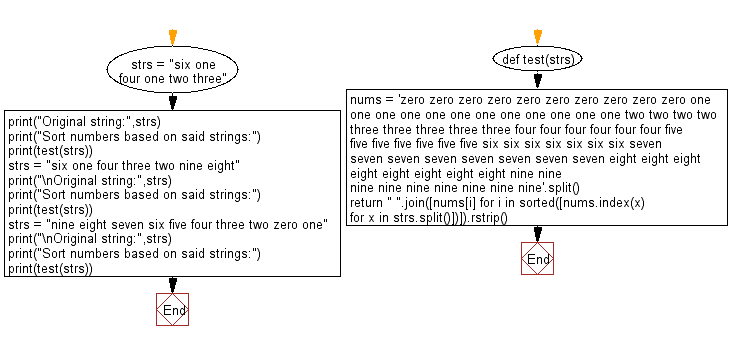﻿ Python: Sort numbers based on strings - w3resource# Python: Sort numbers based on strings

## Python Programming Puzzles: Exercise-41 with Solution

Write a Python program to sort numbers based on strings.

```Input:  six one four one two three
Output:
one two three four six

Input:  six one four three two nine eight
Output:
one two three four six eight nine

Input:  nine eight seven six five four three two  one
Output:
one two three four five six seven eight nine
```

Pictorial Presentation:Sample Solution-1:

Python Code:

``````#License: https://bit.ly/3oLErEI

def test(strs):
return ' '.join([x for x in 'one two three four five six seven eight nine'.split() if x in strs])
strs = "six one four one two three"
print("Original string:",strs)
print("Sort numbers based on said strings:")
print(test(strs))
strs = "six one four three two nine eight"
print("\nOriginal string:",strs)
print("Sort numbers based on said strings:")
print(test(strs))
strs = "nine eight seven six five four three two  one"
print("\nOriginal string:",strs)
print("Sort numbers based on said strings:")
print(test(strs))
``````

Sample Output:

```Original string: six one four one two three
Sort numbers based on said strings:
one two three four six

Original string: six one four three two nine eight
Sort numbers based on said strings:
one two three four six eight nine

Original string: nine eight seven six five four three two  one
Sort numbers based on said strings:
one two three four five six seven eight nine
```

Flowchart:## Visualize Python code execution:

The following tool visualize what the computer is doing step-by-step as it executes the said program:

Sample Solution-2:

Python Code:

``````#License: https://bit.ly/3oLErEI

def test(strs):
nums = 'zero zero zero zero zero zero zero zero zero zero one one one one one one one one one one one one two two two two three three three three three four four four four four four five five five five five five five six six six six six six six seven seven seven seven seven seven seven seven eight eight eight eight eight eight eight eight nine nine nine nine nine nine nine nine nine'.split()
return " ".join([nums[i] for i in sorted([nums.index(x) for x in strs.split()])]).rstrip()

strs = "six one four one two three"
print("Original string:",strs)
print("Sort numbers based on said strings:")
print(test(strs))
strs = "six one four three two nine eight"
print("\nOriginal string:",strs)
print("Sort numbers based on said strings:")
print(test(strs))
strs = "nine eight seven six five four three two zero one"
print("\nOriginal string:",strs)
print("Sort numbers based on said strings:")
print(test(strs))
``````

Sample Output:

```Original string: six one four one two three
Sort numbers based on said strings:
one one two three four six

Original string: six one four three two nine eight
Sort numbers based on said strings:
one two three four six eight nine

Original string: nine eight seven six five four three two zero one
Sort numbers based on said strings:
zero one two three four five six seven eight nine
```

Flowchart:## Visualize Python code execution:

The following tool visualize what the computer is doing step-by-step as it executes the said program:

Python Code Editor :

Have another way to solve this solution? Contribute your code (and comments) through Disqus.

What is the difficulty level of this exercise?

Test your Programming skills with w3resource's quiz.

﻿

## Python: Tips of the Day

Clamps num within the inclusive range specified by the boundary values x and y:

Example:

```def tips_clamp_num(num,x,y):
return max(min(num, max(x, y)), min(x, y))
print(tips_clamp_num(2, 4, 6))
print(tips_clamp_num(1, -1, -6))
```

Output:

```4
-1
```